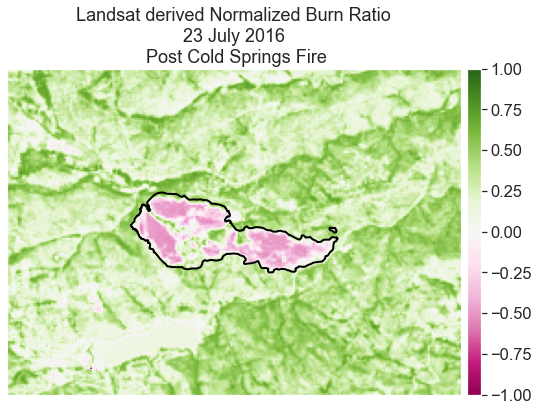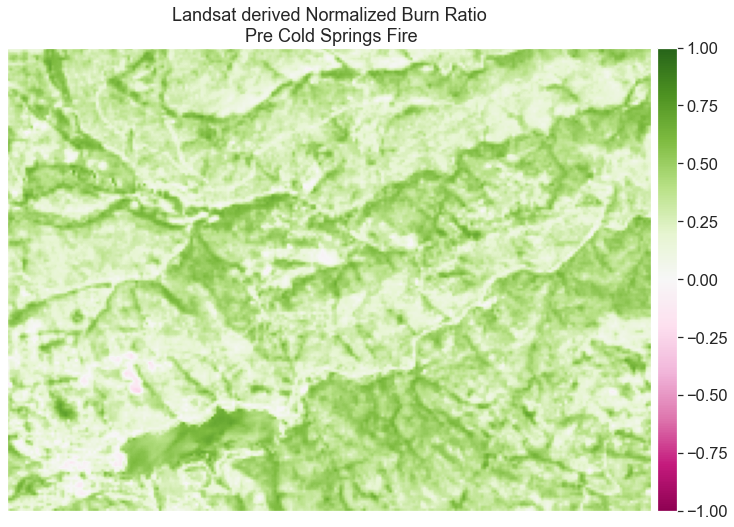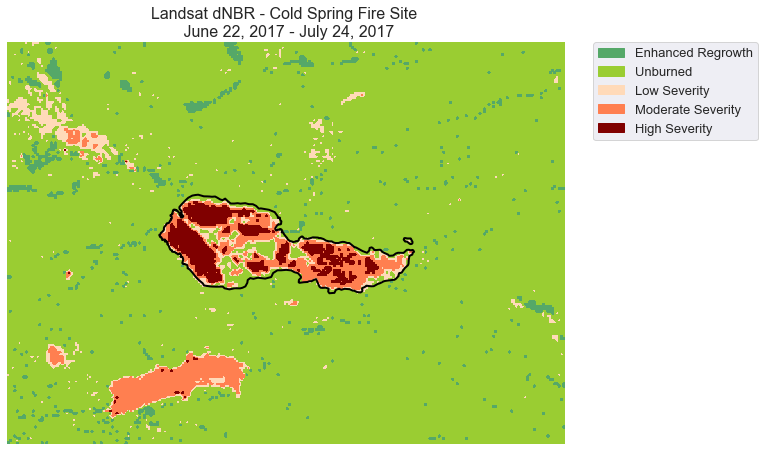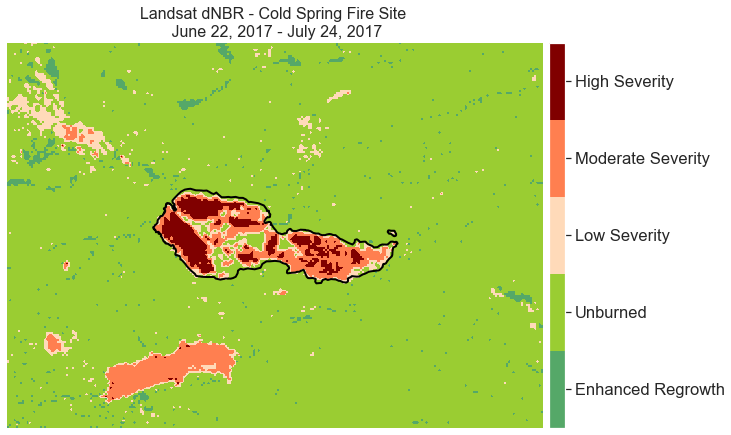# Lesson 3. Calculate and Plot Difference Normalized Burn Ratio (dNBR) using Landsat 8 Remote Sensing Data in Python

## Learning Objectives

• Calculate dNBR in Python.

As discussed in the previous lesson, you can use dNBR to map the extent and severity of a fire. In this lesson, you learn how to create NBR using Landsat data.

You calculate dNBR using the following steps:

1. Open up pre-fire data and calculate NBR
2. Open up the post-fire data and calculate NBR
3. Calculate dNBR (difference NBR) by subtracting post-fire NBR from pre-fire NBR (NBR pre - NBR post fire).
4. Classify the dNBR raster using the classification table.

## Calculate dNBR Using Landsat Data

import os
from glob import glob
import matplotlib.pyplot as plt
from matplotlib import patches as mpatches
from matplotlib.colors import ListedColormap
from matplotlib import colors
import seaborn as sns
import numpy as np
from shapely.geometry import mapping, box
import rasterio as rio
from rasterio.plot import plotting_extent
import geopandas as gpd
import earthpy as et
import earthpy.spatial as es
import earthpy.plot as ep

# Prettier plotting with seaborn
sns.set_style('white')
sns.set(font_scale=1.5)

data1 = et.data.get_data('cold-springs-fire')
data2 = et.data.get_data('cs-test-landsat')
os.chdir(os.path.join(et.io.HOME, 'earth-analytics'))

/opt/conda/lib/python3.8/site-packages/rasterio/plot.py:260: SyntaxWarning: "is" with a literal. Did you mean "=="?
if len(arr.shape) is 2:


To calculate difference Normalized Burn Ratio (dNBR), you first need to calculate NBR for the pre and post fire data. This, of course, presumes that you have data before and after the area was burned from the same remote sensing sensor. Ideally, this data also does not have clouds covering the fire area.

Open up and stack the Landsat post-fire data.

# Import and stack post fire Landsat data
all_landsat_bands_path = os.path.join("data",
"cold-springs-fire",
"landsat_collect",
"LC080340322016072301T1-SC20180214145802",
"crop",
"*band*.tif")

all_landsat_bands = glob(all_landsat_bands_path)
all_landsat_bands.sort()

landsat_post_fire_path = os.path.join("data",
"cold-springs-fire",
"outputs",
"landsat_post_fire.tif")

landsat_post_fire, landsat_post_fire_meta = es.stack(all_landsat_bands,
landsat_post_fire_path)

# Get the plot extent
landsat_extent = plotting_extent(landsat_post_fire,
landsat_post_fire_meta["transform"])

# Open fire boundary layer and reproject it to match the Landsat data
fire_boundary_path = os.path.join("data",
"cold-springs-fire",
"vector_layers",
"fire-boundary-geomac",
"co_cold_springs_20160711_2200_dd83.shp")

# If the CRS are not the same, be sure to reproject
fire_bound_utmz13 = fire_boundary.to_crs(landsat_post_fire_meta['crs'])


Next, you can calculate NBR on the post fire data. Remember that NBR uses different bands than NDVI but the calculation formula is the same. For landsat 8 data you will be using bands 7 and 5. And remember because python starts counting at 0 (0-based indexing), that will be bands 6 and 4 when you access them in your numpy array.

Below the es.normalized_diff() function is used to calculate NBR. You can also calculate NBR like you did NDVI with raster math :

landsat_postfire_nbr = (
landsat_post_fire-landsat_post_fire) / (landsat_post_fire+landsat_post_fire)

# Calculate NBR & plot
landsat_postfire_nbr = es.normalized_diff(
landsat_post_fire, landsat_post_fire)

fig, ax = plt.subplots(figsize=(12, 6))

ep.plot_bands(landsat_postfire_nbr,
cmap='PiYG',
vmin=-1, vmax=1,
extent=landsat_extent,
title="Landsat derived Normalized Burn Ratio\n 23 July 2016 \n Post Cold Springs Fire",
ax=ax,
scale=False)

fire_bound_utmz13.plot(ax=ax, color='None',
edgecolor='black', linewidth=2)
plt.show()Normalized burn ratio (NBR) calculated for the post-Cold Springs fire image for July 23, 2016 from Landsat.

Next, calculate NBR for the pre-fire data. Note that you will have to download the data that is being used below from Earth Explorer following the lessons in this tutorial series.

Also note that you will need to clip or crop the data so that you can subtract the post fire data from the pre fire data. The code to do this is hidden but you did this last week so you should know what to do!

Next you can:

1. calculate NBR on the pre-fire data and then
2. calculate difference dNBR by subtracting the post fire data FROM the pre (pre-post)

The code for this is hidden below because you know how to do this!Normalized burn ratio (NBR) calculated for the pre-Cold Springs fire image from Landsat.

Finally, calculate the difference between the two rasters to calculate the Difference Normalized Burn Ratio (dNBR). Remember to subtract post fire from pre fire.

# Calculate dnbr
dnbr_landsat = nbr_landsat_pre_fire - landsat_postfire_nbr


Finally you can classify the data. Remember that dNBR has a set of classification bins and associated categories that are commonly used. When you have calculated NBR - classify the output raster using the np.digitize() function. Use the dNBR classes below.

SEVERITY LEVEL dNBR RANGE
Enhanced Regrowth > -.1
Unburned -.1 to + .1
Low Severity +.1 to +.27
Moderate Severity +.27 to +.66
High Severity > +.66

NOTE: your min and max values for NBR may be slightly different from the table shown above. In the code example above, np.inf is used to indicate any values larger than .66.

The code to classify below is hidden! You learned how to classify rasters in week 2 of this course.

Data Tip: You learned how to classify rasters in the lidar raster lessons

Finally you are ready to plot your data. Note that legends in python can be tricky so you are provided with two legend options below. In the first plot, you create a legend with individual boxes. Creating a legend this way forces you to use matplotlib patches. Effectively you are drawing each box to create a legend from colors you’ve used in your image.

dnbr_cat_names = ["Enhanced Regrowth",
"Unburned",
"Low Severity",
"Moderate Severity",
"High Severity"]

nbr_colors = ["g", "yellowgreen",
"peachpuff", "coral", "maroon"]
nbr_cmap = ListedColormap(nbr_colors)


Once you setup the legend with

1. a ListedColormap: this is a discrete colormap. Thus it will only contain n colors rather than a gradient of colors.
2. A list of titles for each category in your classification scheme: this is what will be “written” on your legend

You are ready to plot. Below you add a custom legend with unique boxes for each class. This is one way to create a legend. It requires a bit more knowledge of matplotlib under the hood to make this work!

# Plot the data with a custom legend
fig, ax = plt.subplots(figsize=(10, 8))

im = ax.imshow(dnbr_landsat_class,
cmap=nbr_cmap,
extent=landsat_extent)

fire_bound_utmz13.plot(ax=ax, color='None',
edgecolor='black',
linewidth=2)

values = np.unique(dnbr_landsat_class.ravel())

nbr_colors = [im.cmap(im.norm(value)) for value in values]
patches = [mpatches.Patch(color=nbr_colors[i],
label="{l}".format(l=dnbr_cat_names[i])) for i in range(len(dnbr_cat_names))]

plt.legend(handles=patches, bbox_to_anchor=(1.05, 1),
prop={'size': 13})

ax.set_title("Landsat dNBR - Cold Spring Fire Site \n June 22, 2017 - July 24, 2017",
fontsize=16)

# turn off ticks
ax.set_axis_off()
plt.show()Classified difference normalized burn ratio (dNBR) calculated for the Cold Springs fire images from Landsat, with legend created using matplotlib.

Alternatively, you can create the legend with the earthpy.

# Plot the data with a custom legend
fig, ax = plt.subplots(figsize=(10, 8))
im = ax.imshow(dnbr_landsat_class,
cmap=nbr_cmap,
extent=landsat_extent)

fire_bound_utmz13.plot(ax=ax, color='None',
edgecolor='black',
linewidth=2)

values = np.unique(dnbr_landsat_class.ravel())

ep.draw_legend(im,
classes=values,
titles=dnbr_cat_names)

ax.set_title("Landsat dNBR - Cold Spring Fire Site \n June 22, 2017 - July 24, 2017",
fontsize=16)

# turn off ticks
ax.set_axis_off()
plt.show()Classified difference normalized burn ratio (dNBR) calculated for the Cold Springs fire images from Landsat, with legend created using earthpy.

### Create a Colorbar Legend

You can also create a discrete colorbar with labels. This method might be a bit less technical to follow. You can decide what type of legend you prefer for your homework.

# Grab raster unique values (classes)
values = np.unique(dnbr_landsat_class).tolist()

# Add another index value because for n categories
# you need n+1 values to create bins
values =  + values

# Make a color map of fixed colors
nbr_colors = ["g", "yellowgreen", "peachpuff", "coral", "maroon"]
nbr_cmap = ListedColormap(nbr_colors)

# But the goal is the identify the MIDDLE point
# of each bin to create a centered tick
bounds = [((a + b) / 2) for a, b in zip(values[:-1], values[1::1])] + [5.5]

# Define normalization
norm = colors.BoundaryNorm(bounds, nbr_cmap.N)


Then, plot the data but this time add a colorbar rather than a custom legend.

# Plot the data
fig, ax = plt.subplots(figsize=(10, 8))

im = ax.imshow(dnbr_landsat_class,
cmap=nbr_cmap,
extent=landsat_extent,
norm=norm)

fire_bound_utmz13.plot(ax=ax,
color='None',
edgecolor='black',
linewidth=2)

cbar = ep.colorbar(im)

cbar.set_ticks([np.unique(dnbr_landsat_class)])
cbar.set_ticklabels(dnbr_cat_names)
ax.set_title("Landsat dNBR - Cold Spring Fire Site \n June 22, 2017 - July 24, 2017",
fontsize=16)

# Turn off ticks
ax.set_axis_off()
plt.show()Classified difference normalized burn ratio (dNBR) calculated for the Cold Springs fire images from Landsat, with a color bar legend.

## Calculate Total Area of Burned Area

Once you have classified your data, you can calculate the total burn area.

For this lesson, you are comparing the area calculated using modis that is “high severity” to that of Landsat.

You can calculate this using a loop, a function or manually - like so:

# To calculate area, multiply:
# number of pixels in each bin by image resolution
# Result will be in total square meters
landsat_pixel = landsat_pre.res * landsat_pre.res
burned_landsat = (dnbr_landsat_class[dnbr_landsat_class == 5]).size
burned_landsat = np.multiply(burned_landsat, landsat_pixel)

print("Landsat Severe Burn Area:", burned_landsat)

Landsat Severe Burn Area: 711900.0